Chapter 11. Solutions

# Colligative Properties of Ionic Solutes

Learning Objectives

1. Determine the colligative properties of solutions of ionic solutes.

In the section “Colligative Properties of Solutions”, we considered the colligative properties of solutions with molecular solutes. What about solutions with ionic solutes? Do they exhibit colligative properties?

There is a complicating factor: ionic solutes separate into ions when they dissolve. This increases the total number of particles dissolved in solution and increases the impact on the resulting colligative property. Historically, this greater-than-expected impact on colligative properties was one main piece of evidence for ionic compounds separating into ions (increased electrical conductivity was another piece of evidence).

For example, when NaCl dissolves, it separates into two ions:

NaCl(s) → Na+(aq) + Cl(aq)

This means that a 1 M solution of NaCl actually has a net particle concentration of 2 M. The observed colligative property will then be twice as large as expected for a 1 M solution.

It is easy to incorporate this concept into our equations to calculate the respective colligative property. We define the van’t Hoff factor (i) as the number of particles each solute formula unit breaks apart into when it dissolves. Previously, we have always tacitly assumed that the van’t Hoff factor is simply 1. But for some ionic compounds, i is not 1, as shown in Table 11.4 “Ideal van’t Hoff Factors for Ionic Compounds”.

Table 11.4 Ideal van’t Hoff Factors for Ionic Compounds
Compound i
NaCl 2
KBr 2
LiNO3 2
CaCl2 3
Mg(C2H3O2)2 3
FeCl3 4
Al2(SO4)3 5

The ideal van’t Hoff factor is equal to the number of ions that form when an ionic compound dissolves.

Example 11.17

Predict the van’t Hoff factor for Sr(OH)2.

Solution
When Sr(OH)2 dissolves, it separates into one Sr2+ ion and two OH ions:

Sr(OH)2 → Sr2+(aq) + 2OH(aq)

Because it breaks up into three ions, its van’t Hoff factor is 3.

Test Yourself
What is the van’t Hoff factor for Fe(NO3)3?

4

It is the “ideal” van’t Hoff factor because this is what we expect from the ionic formula. However, this factor is usually correct only for dilute solutions (solutions less than 0.001 M). At concentrations greater than 0.001 M, there are enough interactions between ions of opposite charge that the net concentration of the ions is less than expected—sometimes significantly. The actual van’t Hoff factor is thus less than the ideal one. Here, we will use ideal van’t Hoff factors.

Revised equations to calculate the effect of ionization are then easily produced: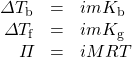where all variables have been previously defined. To calculate vapour pressure depression according to Raoult’s law, the mole fraction of solvent particles must be recalculated to take into account the increased number of particles formed on ionization.

Example 11.18

Determine the freezing point of a 1.77 m solution of NaCl in H2O.

Solution
For NaCl, we need to remember to include the van’t Hoff factor, which is 2. Otherwise, the calculation of the freezing point is straightforward: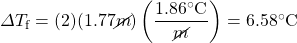This represents the change in the freezing point, which is decreasing. So we have to subtract this change from the normal freezing point of water, 0.00°C: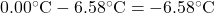Test Yourself
Determine the boiling point of a 0.887 m solution of CaCl2 in H2O.

101.36°C

# Food and Drink App: Salting Pasta Cooking Water

When cooking dried pasta, many recipes call for salting the water before cooking the pasta. Some argue — with colligative properties on their side — that adding salt to the water raises the boiling point, thus cooking the pasta faster. Is there any truth to this?

To judge the veracity of this claim, we can calculate how much salt should be added to the water to raise the boiling temperature by 1.0°C, with the presumption that dried pasta cooks noticeably faster at 101°C than at 100°C (although a 1° difference may make only a negligible change in cooking times). We can calculate the molality that the water should have: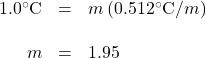We have ignored the van’t Hoff factor in our estimation because this obviously is not a dilute solution. Let us further assume that we are using 4 L of water (which is very close to 4 qt, which in turn equals 1 gal). Because 4 L of water is about 4 kg (it is actually slightly less at 100°C), we can determine how much salt (NaCl) to add: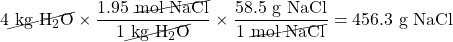This is just over 1 lb of salt and is equivalent to nearly 1 cup in the kitchen. In your experience, do you add almost a cup of salt to a pot of water to make pasta? Certainly not! A few pinches, perhaps one-fourth of a teaspoon, but not almost a cup! It is obvious that the little amount of salt that most people add to their pasta water is not going to significantly raise the boiling point of the water.

So why do people add some salt to boiling water? There are several possible reasons, the most obvious of which is taste: adding salt adds a little bit of salt flavour to the pasta. It cannot be much because most of the salt remains in the water, not in the cooked pasta. However, it may be enough to detect with our taste buds. The other obvious reason is habit; recipes tell us to add salt, so we do, even if there is little scientific or culinary reason to do so.

Key Takeaways

• For ionic solutes, the calculation of colligative properties must include the fact that the solutes separate into multiple particles when they dissolve.
• The equations for calculating colligative properties of solutions of ionic solvents include the van’t Hoff factor, i.

Exercises

# Questions

1. Explain why we need to consider a van’t Hoff factor for ionic solutes but not for molecular solutes.
2. NaCl is often used in winter to melt ice on roads and sidewalks, but calcium chloride (CaCl2) is also used. Which would be better (on a mole-by-mole basis), and why?
3. Calculate the boiling point of an aqueous solution of NaNO3 made by mixing 15.6 g of NaNO3 with 100.0 g of H2O. Assume an ideal van’t Hoff factor.
4. Many labs use a cleaning solution of KOH dissolved in C2H5OH. If 34.7 g of KOH were dissolved in 88.0 g of C2H5OH, what is the boiling point of this solution? The normal boiling point of C2H5OH is 78.4°C and its Kb = 1.19°C/m. Assume an ideal van’t Hoff factor.
5. What is the freezing point of a solution made by dissolving 345 g of CaCl2 in 1,550 g of H2O? Assume an ideal van’t Hoff factor.
6. A classic homemade ice cream can be made by freezing the ice cream mixture using a solution of 250 g of NaCl dissolved in 1.25 kg of ice water. What is the temperature of this ice water? Assume an ideal van’t Hoff factor.
7. Seawater can be approximated as a 3.5% NaCl solution by mass; that is, 3.5 g of NaCl are combined with 96.5 g H2O. What is the osmotic pressure of seawater? Assume an ideal van’t Hoff factor.
8. The osmotic pressure of blood is 7.65 atm at 37°C. If blood were considered a solution of NaCl, what is the molar concentration of NaCl in blood? Assume an ideal van’t Hoff factor.
9. What is the vapour pressure of an aqueous solution of 36.4 g of KBr in 199.5 g of H2O if the vapour pressure of H2O at the same temperature is 32.55 torr? What other solute(s) would give a solution with the same vapour pressure? Assume an ideal van’t Hoff factor.
10. Assuming an ideal van’t Hoff factor, what mole fraction is required for a solution of Mg(NO3)2 to have a vapour pressure of 20.00 torr at 25.0°C? The vapour pressure of the solvent is 23.61 torr at this temperature.

1. Ionic solutes separate into more than one particle when they dissolve, whereas molecular solutes do not.
1. 101.9°C
1. −7.5°C
1. 30.3 atm
1. 30.86 torr; any two-ion salt should have the same effect.